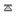## Time and date

Add block >Utilities >Time and date
This function returns the current SERVER time offset by your timezone in various formats
yyyy-mm-dd
<xt:tdate format="Y-m-d" tzone="0" />

2023-12-04
hh:mm
<xt:tdate format="H:i" tzone="0" />

07:35
yyyy-m-dd hh:mm:ss
<xt:tdate format="Y-m-d H:i:s" tzone="0" />

2023-12-04 07:35:53
yy/mm/dd
<xt:tdate format="y/m/d" tzone="0" />

23/12/04
dd/mm/yy
<xt:tdate format="d/m/y" tzone="0" />

04/12/23
mm/dd/yy
<xt:tdate format="m/d/y" tzone="0" />

12/04/23
 LIST OF FORMAT OPTIONS A = AM/PM (AM) a=am/pm (am) B = Swatch internet time (358) c = full (2023-12-04T07:35:53+00:00) D = day of week (Mon) d = day of month (04) e = timezone (GMT) F = name of month (December) g = hour (7) (12 hr no leading 0) h = hour (07) (12hr leading 0) G = hour (7) (24hr no leading 0) H = hour (07) (24hr leading 0) i = minutes (35) I = daylight saving (0) (1=yes 0=no) j = day of month (4) (no leading 0) l = day of week (Monday) L = leapyear (1=yes 0=no) (0) M = name of month (Dec) m = month (12) (leading 0) n = month (12) (no leading 0) N = day (1=mon 7=sun) (1) w = day (sun=0 sat=6) (1) r = full (Mon, 04 Dec 2023 07:35:53 +0000) S = type of date (1st 2nd 3rd 4th) (th) s = seconds (53) T = timezone abbrv (GMT) t = days in month (31) U = seconds since 1/1/1970 (1701675353) W = week number (49) y = year (23) Y = year (2023) z = days (0=jan 1) (337) O = difference from GMT in hours (+0000) P = same with a colon (+00:00) u = Microseconds (000000)About us Contact Forum